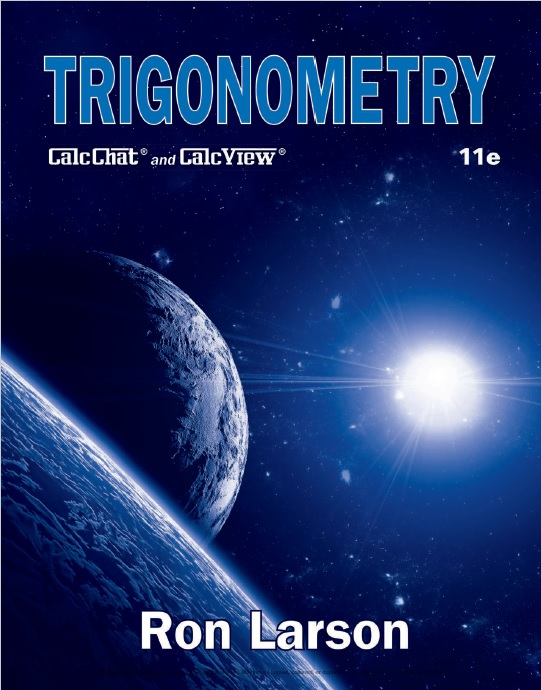# Trigonometry with CalcChat and CalcView, 11th Edition PDF by Ron Larson

## Trigonometry with CalcChat and CalcView, 11th Edition

By Ron LarsonContents:

Prerequisites 1

P.1 Review of Real Numbers and Their Properties 2

P.2 Solving Equations 14

P.3 The Cartesian Plane and Graphs of Equations 26

P.4 Linear Equations in Two Variables 40

P.5 Functions 53

P.6 Analyzing Graphs of Functions 67

P.7 A Library of Parent Functions 78

P.8 Transformations of Functions 85

P.9 Combinations of Functions: Composite Functions 94

P.10 Inverse Functions 102

Summary and Study Strategies 111

Review Exercises 114

Chapter Test 117

Proofs in Mathematics 118

P.S. Problem Solving 119

Trigonometry 121

1.1 Radian and Degree Measure 122

1.2 Trigonometric Functions: The Unit Circle 132

1.3 Right Triangle Trigonometry 139

1.4 Trigonometric Functions of Any Angle 150

1.5 Graphs of Sine and Cosine Functions 159

1.6 Graphs of Other Trigonometric Functions 170

1.7 Inverse Trigonometric Functions 180

1.8 Applications and Models 190

Summary and Study Strategies 200

Review Exercises 202

Chapter Test 205

Proofs in Mathematics 206

P.S. Problem Solving 207

Analytic Trigonometry 209

2.1 Using Fundamental Identities 210

2.2 Verifying Trigonometric Identities 217

2.3 Solving Trigonometric Equations 224

2.4 Sum and Difference Formulas 236

2.5 Multiple-Angle and Product-to-Sum Formulas 243

Summary and Study Strategies 252

Review Exercises 254

Chapter Test 256

Proofs in Mathematics 257

P.S. Problem Solving 259

3.1 Law of Sines 262

3.2 Law of Cosines 271

3.3 Vectors in the Plane 278

3.4 Vectors and Dot Products 291

Summary and Study Strategies 300

Review Exercises 302

Chapter Test 305

Cumulative Test for Chapters 1–3 306

Proofs in Mathematics 308

P.S. Problem Solving 311

Complex Numbers 313

4.1 Complex Numbers 314

4.2 Complex Solutions of Equations 321

4.3 The Complex Plane 329

4.4 Trigonometric Form of a Complex Number 336

4.5 DeMoivre’s Theorem 342

Summary and Study Strategies 348

Review Exercises 350

Chapter Test 353

Proofs in Mathematics 354

P.S. Problem Solving 355

Exponential and Logarithmic Functions 357

5.1 Exponential Functions and Their Graphs 358

5.2 Logarithmic Functions and Their Graphs 369

5.3 Properties of Logarithms 379

5.4 Exponential and Logarithmic Equations 386

5.5 Exponential and Logarithmic Models 396

Summary and Study Strategies 408

Review Exercises 410

Chapter Test 413

Proofs in Mathematics 414

P.S. Problem Solving 415

Topics in Analytic Geometry 417

6.1 Lines 418

6.2 Introduction to Conics: Parabolas 425

6.3 Ellipses 434

6.4 Hyperbolas 443

6.5 Rotation of Conics 453

6.6 Parametric Equations 461

6.7 Polar Coordinates 471

6.8 Graphs of Polar Equations 477

6.9 Polar Equations of Conics 485

Summary and Study Strategies 492

Review Exercises 494

Chapter Test 497

Cumulative Test for Chapters 4–6 498

Proofs in Mathematics 500

P.S. Problem Solving 503

Appendix A

Concepts in Statistics (online)*

A.1 Representing Data

A.2 Analyzing Data

A.3 Modeling Data

Answers to Odd-Numbered Exercises and Tests A1

Index A79

Index of Applications (online)*

*Available at the text companion website LarsonPrecalculus.com

This book is US\$10
To get free sample pages OR Buy this book
Send email: [email protected]